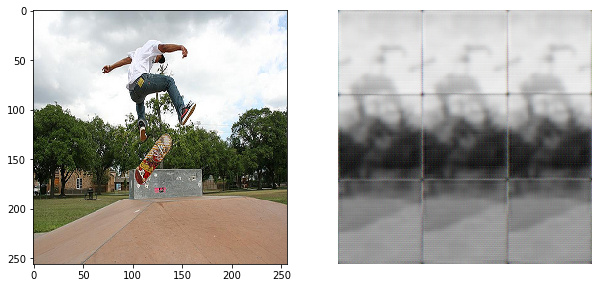# Image segmentation on autoencoder output

I have an autoencoder NN:

``````class Autoencoder(nn.Module):
def __init__(self):
super(Autoencoder, self).__init__()

self.Encoder = nn.Sequential(
nn.LeakyReLU(0.1),
nn.MaxPool2d(kernel_size = (2,2)),
###Now 128*128*64
nn.LeakyReLU(0.1),
nn.MaxPool2d(kernel_size = (2,2)),
###Now 64*64*32
nn.LeakyReLU(0.1),
nn.MaxPool2d(kernel_size = (2,2)),
###Now 32*32*16
#nn.Conv2d(16,16,kernel_size = (10,10),padding_mode = 'same',bias = True),
#nn.LeakyReLU(0.1),
#nn.MaxPool2d(kernel_size = (2,2)))
)
###Now 16*16*16

self.Decoder = nn.Sequential(
#nn.ConvTranspose2d(16,16,kernel_size = (3,3)),
#nn.LeakyReLU(0.1),
nn.ConvTranspose2d(32,64,kernel_size = (3,3),stride = 2, padding = 1),
nn.LeakyReLU(0.1),
nn.ConvTranspose2d(64,64,kernel_size = (6,6),stride = 2,padding = 1),
nn.LeakyReLU(0.1),
nn.ConvTranspose2d(64,3,kernel_size = (8,8), stride = 2, padding = 3),
nn.Tanh())

def forward(self,x,corruption):
x = self.Encoder(x)
#print('Encoder output: ',x.shape)
if x.shape == corruption.shape:
x = x * corruption
else:
corruption = corruption[0:len(x)]
x = x * corruption
x = self.Decoder(x)
#print('Decoder output: ',x.shape)
return x
``````

But i have a problem, where output images of 2562563 are segmented into 3x3 grid even after some learning (it also appeared on simplier models)
looking at results after first epoch, i can make a theory on what happens, but i still cant understand how to fix it:It looks like something splits images into top-middle-bottom fashion and then replicates it three times, but what is doing it, i am not sure

Full code available here: